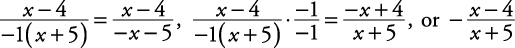## Simplifying Rational Expressions

To simplify a rational expression:

1. Completely factor numerators and denominators.

2. Reduce common factors.

##### Example 1

Simplify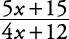.##### Example 2

Simplify.Whenever possible, try to write all polynomials in descending order with a positive leading coefficient. To have a positive leading coefficient, occasionally –1 has to be factored out of the polynomial.

##### Example 3

Simplify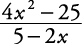.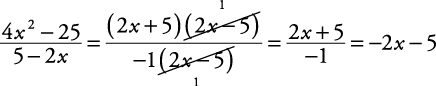##### Example 4

Simplify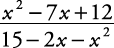.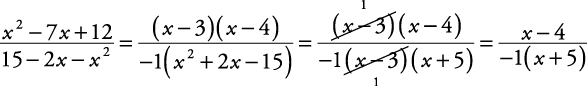This answer can be expressed in other ways.